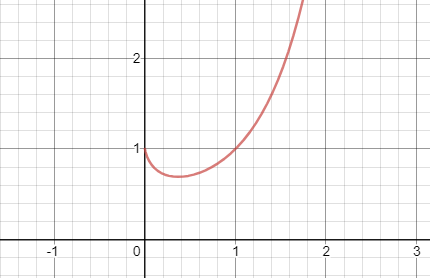# 1 to the 0 power

• So I was on Khan Academy math, and they told me that any number to the 0 power is 1 because normal exponents like 2 to the second power can also be expressed as 1 x (2 x 2), and so any number to the zero power would just be that 1 left over.

But personally, I feel like something is wrong with this. Exponents are how many times a number is multiplied by, right? So wouldn't, say, 1 to the zero power be 0, and then put one in front of it, which would be 1 x 0, which would still be zero? It feels like with exponents of 0, we're not being consistent.

On a material level, it's the same thing. I have 0 copies of some amount of some thing, so I have one copy of it? Isn't mathematics created so we can use it in the real world?

I’m not an expert myself, but I’ll try to answer your question.
First, let’s look at $$1 \times 0$$. $$0$$, right?
Why is the answer $$0$$?
Multiplication can be thought repeated addition, and 1 added to itself $$0$$ times is 0.
But is there anything interesting about $$0$$?
Anything $$+0$$ is always going to be equal to itself. I think this is called the “addition identity”.
Now, let’s look at $$1^0$$. The answer is $$1$$.
But why $$1$$?
First, exponents or powers can be thought as repeated multiplication.
$$1$$ is what we call the “multiplication identity”, as anything $$\times 1$$ is always equal to itself.
So my assumption is that mathematicians continued this pattern of “anything to the operation of $$0$$ is equal to the identity of the operation in question’s definition, which is another operation repeated.”
This is a bit long, so I hope it doesn’t waste too much time reading this post.
Hope it helps!• @RZ923 That is an excellent answer!I'd like to add another comment here which comes from a different angle:

@The-Rogue-Blade said in 1 to the 0 power:

But personally, I feel like something is wrong with this. Exponents are how many times a number is multiplied by, right? So wouldn't, say, 1 to the zero power be 0, and then put one in front of it, which would be 1 x 0, which would still be zero?

As an example of exponent multiplication, when we times together $$2^3$$ and $$2^4,$$ for example, then we get

$$2^3 \times 2^4 = 2^7$$

since

$$\textcolor{blue}{2 \times 2 \times 2} \times \textcolor{red}{2 \times 2 \times 2 \times 2} = 2 \times 2 \times 2 \times 2 \times 2 \times 2 \times 2$$

$$\textcolor{blue}{2^3} \times \textcolor{red}{2^4} = 2^{3 + 4}$$

If we multiply something to the zero power, like $$2^0,$$ and another power of $$2,$$

$$2^0 \times 2^4 = 2^{0 + 4}$$

we will expect the power on the exponent $$2^4$$ to remain the same, since $$0$$ is the identity under addition. (This relates to what @RZ923 said, about additive identities.)

Thus $$2^0$$ is the multiplicative identity, and it's because when the powers sum together, $$0$$ is summed as the additive identity.

$$2^0 \times 2^{\textcolor{red}{\text{anything}}} = 2^{0 + \textcolor{red}{\text{anything}}}$$

$$2^0 \times 2^{\textcolor{red}{\text{anything}}} = 2^{\textcolor{red}{\text{anything}}}$$

$$1 \times 2^{\textcolor{red}{\text{anything}}} = 2^{\textcolor{red}{\text{anything}}}$$

$$\boxed{1 = 2^0}$$• I mean, there's also like continuing a pattern, but shouldn't math be applied and not just working in theory?

• We already have a ton of exceptions in math, and including one here wouldn't be a problem. Besides, it would be possible to derive this exception easily.

• @The-Rogue-Blade Good point! In practice, $$x^0 = 1$$ works in the sense that it's better than $$x^0 = 0,$$ because if $$x^0 = 0,$$ then we get

$$\textcolor{red}{x^0} \times x^4 = 0$$

$$\textcolor{red}{0} \times x^4 = 0$$

which is a bit strange, since $$\textcolor{red}{x^0}$$ would be able to have this destructive property of obliterating all other exponents.Additionally, when we prime factorize a number, like $$60,$$

$$60 = 2^2 \times 3^1 \times 5^1$$

we might want to be able to list them out with all primes as a sort of basis, like

$$60 = 2^2 \times 3^1 \times 5^1 \times \textcolor{red}{7^0} \times \textcolor{red}{11^0} \times \textcolor{red}{13^0} \ldots$$

We would only be able to do so if we define $$x^0$$ to equal $$1.$$

• Well, that's just my point of view on this. I was also about to ask why the code was weird• @The-Rogue-Blade It might be because it's a fresh post, and it takes awhile for the site to render the code...? I'm not sure, but refreshing often fixes the problem!• Interesting discussion! What do you guys think $$0^0$$ is?

• @thomas It's probably undefined. Since 0^x = 0 and x^0=1 for all nonzero x, if x were to be 0, we would have 0=1, which is very, uh,





let's just not think about that.

• @Potato2017 Interesting! It seems like it's unclear if we can even do it at all...

Would perhaps this graph change your mind?This is a graph of the function $$x^x$$. What this graph is saying is that if you look at $$1^1$$, $$0.1^{0,1}$$, $$0.01^{0.01}$$, ... these numbers get closer and closer to $$1$$. Do you think that means that $$0^0$$ should be $$1$$? Why or why not?

I came up with another way of explaining why $$1^0$$ is $$1$$. It had something to do with Place Value.
Any number, say $$1729$$, can be represented with powers of 10.
For example, the $$1$$ represents $$1000$$, or $$1 \times 10^3$$. The $$7$$ represents $$7 \times 10^2$$, and the $$2$$ represents $$2 \times 10^1$$.
Now let’s look at the $$9$$. What does it represent.
Continuing our pattern, it represents $$9 \times 10^0$$.
So $$10^0$$ must be one. If it’s $$0$$, then we don’t have place value.
Hope that helps•